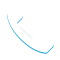### IBM SPSS Modeler Foundations (V18.2) – 0A069G CR

Details

Course Code: 0A069G

Brand: DS&BA – SPSS

Category: Analytics

Skill Level: Basic

Duration: 16.0H

Modality: CR

Audience

• Data scientists
• Clients who are new to IBM SPSS Modeler or want to find out more about using it

Prerequisites

Short Summary

This course teaches how to use IBM SPSS Modeler 18.2.

Overview

This course provides the foundations of using IBM SPSS Modeler and introduces the participant to data science. The principles and practice of data science are illustrated using the CRISP-DM methodology. The course provides training in the basics of how to import, explore, and prepare data with IBM SPSS Modeler v18.2, and introduces the student to modeling.

Topic

Introduction to IBM SPSS Modeler
• Introduction to data science
• Describe the CRISP-DM methodology
• Introduction to IBM SPSS Modeler
• Build models and apply them to new data

Collect initial data
• Describe field storage
• D
escribe field measurement level
• Import from various data formats
• Export to various data formats

Understand the data
• Audit the data
• Check for invalid values
• Take action for invalid values
• Define blanks

Set the unit of analysis
• Remove duplicates
• Aggregate data
• Transform nominal fields into flags
• Restructure data

Integrate data
• Append datasets
• Merge datasets
• Sample records

Transform fields
• Use the Control Language for Expression Manipulation
• Derive fields
• Reclassify fields
• Bin fields

Further field transformations
• Use functions
• Replace field values
• Transform distributions

Examine relationships
• Examine the relationship between two categorical fields
• Examine the relationship between a categorical  and continuous field
• Examine the relationship between two continuous fields

Introduction to modeling
• Describe modeling objectives
• Create supervised models
• Create segmentation models

Improve efficiency
• Use database scalability by SQL pushback
• Process outliers and missing values with the Data Audit node
• Use the Set Globals node
• Use parameters
• Use looping and conditional execution

Objectives

Introduction to IBM SPSS Modeler
• Introduction to data science
• Describe the CRISP-DM methodology
• Introduction to IBM SPSS Modeler
• Build models and apply them to new data

Collect initial data
• Describe field stora
ge
• Describe field measurement level
• Import from various data formats
• Export to various data formats

Understand the data
• Audit the data
• Check for invalid values
• Take action for invalid values
• Define blanks

Set the unit of analysis
• Remove duplicates
• Aggregate data
• Transform nominal fields into flags
• Restructure data

Integrate data
• Append datasets
• Merge datasets
• Sample records

Transform fields
• Use the Control Language for Expression Manipulation
• Derive fields
• Reclassify fields
• Bin fields

Further field transformations
• Use functions
• Replace field values
• Transform distributions

Examine relationships
• Examine the relationship between two categorical fields
• Examine the relationship between a categorical and continuous field
• Examine the relationship between two continuous fields

Introduction to modeling
• Describe modeling objectives
• Create supervised models
• Create segmentation models

Improve efficiency
• Use database scalability by SQL pushback
• Process outliers and missing values with the Data Audit node
• Use the Set Globals node
• Use parameters
• Use looping and conditional execution

Tags :Call Now +27 72 266 2599